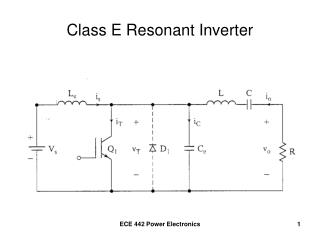Download PresentationClass E Resonant Inverter

# Class E Resonant Inverter - PowerPoint PPT PresentationDownload Presentation## Class E Resonant Inverter

- - - - - - - - - - - - - - - - - - - - - - - - - - - E N D - - - - - - - - - - - - - - - - - - - - - - - - - - -
##### Presentation Transcript

1. Class E Resonant Inverter ECE 442 Power Electronics

2. Mode 1 Operation – Turn Q1 ON at t = 0 Turn Q1 OFF when vo = 0 volts ECE 442 Power Electronics

3. For sinusoidal current, The current through the transistor (switch) The switch is turned OFF when the output voltage becomes = 0, and the current is “transferred” to the branch containing the capacitor. ECE 442 Power Electronics

4. Mode 1 ECE 442 Power Electronics

5. ECE 442 Power Electronics

6. Mode 2 Operation • Q1 is turned OFF • Diode D limits negative switch voltage ECE 442 Power Electronics

7. Capacitor current becomes When the switch current falls to zero, ECE 442 Power Electronics

8. Mode 2 ECE 442 Power Electronics

9. Waveform Summary ECE 442 Power Electronics

10. Example 8.9 • A class E inverter operates at resonance and has VS = 12 Volts and R = 10 Ω. • The switching frequency is 25 kHz. • Determine the optimum values of L, C, Ce, and Le • Use MultiSim to plot the output voltage v0 and the switch voltage vT for k = 0.304. Assume that Q = 7. ECE 442 Power Electronics

11. Optimum Parameters ECE 442 Power Electronics

12. Example 8.9 (continued) ECE 442 Power Electronics

13. ECE 442 Power Electronics

14. Check the damping factor and resonant frequency ECE 442 Power Electronics

15. Example 8.9 ECE 442 Power Electronics

16. Switch Voltage Load Voltage ECE 442 Power Electronics

17. Class E Resonant Rectifier very large for power factor correction ECE 442 Power Electronics

18. Mode 1 Operation -- D1 OFF ECE 442 Power Electronics

19. Mode 2 Operation -- D1 ON ECE 442 Power Electronics

20. D1 switches OFF at 0 volts(0 voltage switching) • When the current iL falls to 0, the diode turns OFF. • When iL falls below Io, C discharges via D1 • At turn-off, iD=iL=0 and vD=vC=0. • The capacitor current, iC=C(dvC/dt)=0, or (dvC/dt) = 0. ECE 442 Power Electronics

21. Waveform Summary iL = iC + iD ECE 442 Power Electronics

22. Example 8.10 • A Class E rectifier supplies a load power of PL=400mW at Vo=4V. The peak supply voltage is Vm=10V. The supply frequency is f=250kHz. The peak-to-peak ripple on the dc output voltage is ΔVo=40mV. • Determine the values of L, C, and Cf. • Determine the rms and dc currents of L and C. ECE 442 Power Electronics

23. Example 8.10 (continued) • Choose C=10nF. • The resonant frequency will be 250kHz. • Details on the following slide ECE 442 Power Electronics

24. ECE 442 Power Electronics

25. ECE 442 Power Electronics

26. Example 8.10 (MultiSim) ECE 442 Power Electronics

27. ECE 442 Power Electronics

28. Load Current 500 mAp-p Load Voltage 50 mVp-p ECE 442 Power Electronics